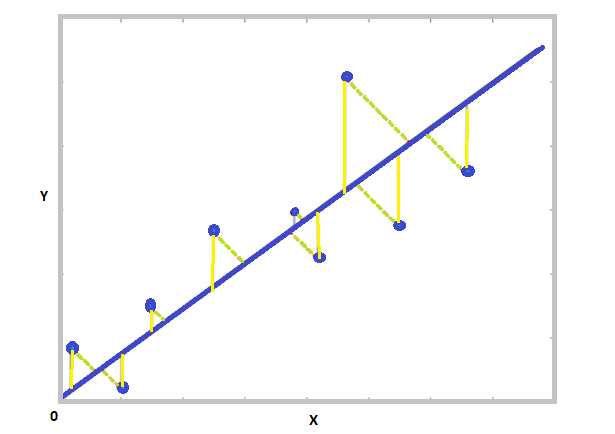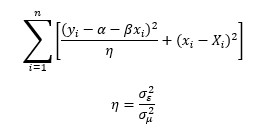# Orthogonal distance regression using SciPy

• Difficulty Level : Expert
• Last Updated : 10 Jan, 2022

Regression basically involves determining the relationship between a dependent variable and one or more independent variables. It generally involves finding the best fit line that minimizes the sum of squared errors for each point. Based on the implementation procedures, regression algorithms are classified as linear regression, ridge regression, lasso regression, polynomial regression, and so on. In this article let us discuss Orthogonal distance regression and see the practical implementation of Orthogonal distance regression in Scipy.

## Orthogonal regression

Unlike normal regression problems where errors are measured vertically with respect to the fitted line, orthogonal distance regression involves in calculating the orthogonal distance of the points with respect to the fitted line, this allows taking into account the errors in measurements for both independent and dependent variables along the x and y-axis, as shown in the figure. This way of calculating the perpendicular distance adds more robustness to the model. Orthogonal Distance regression minimizes the sum of squared perpendicular distances, unlike the sum of least squared distances.Orthogonal regression is generally applied when both Y and X are susceptible to error and can also be applied to the transformable non-linear model. Orthogonal regression assumes that there is a linear relationship between the true values of the dependent and independent variables. The observed values of Y and X have a small error added to them. Given n pairs of measured values, Orthogonal regression involves finding a line that minimized the below equation.Here, ε, μ are errors in the measure value σ denotes the variance of errors.

The orthogonal distance regression is implemented using ODRPACK which is a FORTRAN – 77 based library. The scipy.odr package provides an OOPS interface to ODRPACK.

### Approach

• Import the necessary python packages like numpy, matplotlib, and random.
• Import ODR function from scipy. Create a sample feature and a target array using numpy.
• Based on the distribution of feature variables we can define the target function that has to be used for fitting, by the odr function. Here, we use a simple linear equation as a target function that is used by the odr function to fit the model.
• Pass the defined custom target function to the odr.Model() function. Here the model is fitted.
• Now, transform the feature and target variables by passing them through odr.Data() function.
• Then, pass the transformed feature and target variable and the fitted model along with hyperparameter beta (a small value).
• Use the run() function on the final odr model to compute and print the result

Code:

## Python3

 `# import the necessary python packages``import` `numpy as np``import` `matplotlib.pyplot as plt` `# odr function from scipy package``# is used to perform ODR regression``from` `scipy ``import` `odr ``import` `random as r` `# Create a sample feature array and a target array``feature ``=` `np.array(np.arange(``1``, ``11``))``# shuffle the created array``np.random.shuffle(feature)``# create a target array of random numbers``target ``=` `np.array([``0.65``, ``-``.``75``, ``0.90``, ``-``0.5``, ``0.14``,``                   ``0.84``, ``0.99``, ``-``0.95``, ``0.41``, ``-``0.28``])` `# Define a function (quadratic in our case)``# to fit the data with.``# odr initially assumes a linear function``def` `target_function(p, x):``    ``m, c ``=` `p``    ``return` `m``*``x ``+` `c` `#  model fitting.``odr_model ``=` `odr.Model(target_function)` `# Create a Data object using sample data created.``data ``=` `odr.Data(feature, target)` `# Set ODR with the model and data.``ordinal_distance_reg ``=` `odr.ODR(data, odr_model,``                               ``beta0``=``[``0.2``, ``1.``])` `# Run the regression.``out ``=` `ordinal_distance_reg.run()` `# print the results``out.pprint()`

Output:

```Beta:                    [-0.01059931  0.2032962 ]

Beta Std Error:        [0.08421527 0.52254163]

Beta Covariance:       [[ 0.01212265 -0.06667458]

[-0.06667458  0.46672142]]

Residual Variance:      0.5850379776588954

Inverse Condition #:    0.06924525890982118

Reason(s) for Halting:
Sum of squares convergence```

The odr algorithm will return the beta value, std error, and covariance of beta values which can be used to fit the regression line.

My Personal Notes arrow_drop_up